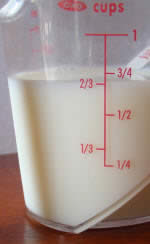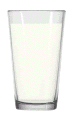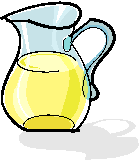# US Standard Volume

These are the most common measurements:

• Fluid Ounces
• Cups
• Pints
• Quarts
• GallonsFluid Ounces (fl oz) are small.

About how much fits in a small medicine cup.

### Fluid Ounce!

"Fluid Ounce" is used for volume, "Ounce" for mass, and they are different.

• For example, 1 fluid ounce of honey has a mass of about 1.5 ounces!
• But for water, 1 fluid ounce has a mass of about 1 ounce.

If you mean an ounce of fluid say "fluid ounce" ("fl oz")## Cups

A small glass holds about 8 fluid ounces, which is also called 1 cup.

1 cup = 8 fluid ounces

Here we have 2/3 cup (two-thirds of a cup) of milk, or about 5 fluid ounces

By the way ... we can measure volume using special Measuring Cups ... !## Pints

A pint is equal to 2 cups (example: a large glass of milk!)

1 pint = 2 cups = 16 fluid ounces

 When measuring many cups of liquid all put together we might want to use quarts. A quart (qt) is the same thing as 4 cups or 2 pints.  1 quart = 2 pints = 4 cups = 32 fluid ouncesIf we still need more liquid we can switch to using gallons. A gallon (gal) is the same as 16 cups or 8 pints or 4 quarts.  It is the largest liquid measurement. (Note that a quart is a quarter of a gallon!)## In Summary:

1 gallon = 4 quarts = 8 pints = 16 cups = 128 fluid ounces## Other Volume Measurements

### Cubic InchVolume is length by length by length,

so the Cubic Inch is a cube that is 1 inch on each side.

The Unit is written many ways, such as:

• in3
• inch3
• cu in
• cu inch
• cubic inches
• etc ...

### Barrel of Oil

1 oil barrel (bbl) is equal to 42 gallons (or about 160 Liters)

## More Examples

A fluid ounce is:

• very close to 30 milliliters (metric volume)
• more precisely 29.5735297 milliliters

A pint is:

• more precisely 473.176473 milliliters

A quart is: